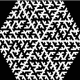Feeds:
Posts

## A Calendar Puzzle

Here’s a nice, neat puzzle for those with a spare minute.

I was at a mate’s house and saw that he had a desk calendar that worked as follows. The day of the month was displayed using two cubes, which had one number on each face. A quick calculation would suggest that the first cube (the tens column) needs the numbers 0,1,2,3. But the second cube (the ones column) needs the ten numbers 0-9. Each cube only has six faces, so it looks like we’re 4 + 10 – 12 = 2 faces short. So how does it work?

### 5 Responses

1. on June 20, 2008 at 5:08 am | ReplyTim McKenzie

Spoiler! (I hope.)

Well, I would be inclined to display the day of the month in base six, but I’m guessing they had something like 0, 1, 2, 3, 4, 5, on one, and 0, 1, 2, 7, 8, 9 on the other. The 9 can double as a 6. First, display the ones digit using the second cube if possible, or the first cube otherwise.

If you successfully displayed the ones digit using the second cube, then display the tens digit (0, 1, 2, or 3) using the first cube.

On the other hand, if you had to use the first cube to display the ones digit, then the day of the month ends in 3, 4, or 5. Since it can’t be the 33rd, 34th, or 35th day of the month, you must be able to display the tens digit (0, 1, or 2) using the second cube.

2. on June 26, 2008 at 11:28 pm | Replydougaj4

The only numbers that will ever need to be repeated are 1 (for the 11th) and 2 (for the 22nd), so put 1 and 2 on both cubes, then 0, 3, 4, 5 on one and 6, 7, 8, 9 on the other. This will allow the display of all numbers up to 32.

3. on June 27, 2008 at 7:58 am | ReplyTim McKenzie

dougaj4: What do you display on the 30th day of the month? Your only 0 and your only 3 are on the same cube.

4. on June 27, 2008 at 10:54 am | Replydougaj4

Good point Tim. Swap the 0 with 6, so you have:
1,2,3,4,5,6
0,1,2,7,8,9

But if you always want to dispay two cubes (i.e. 0x for the first to 9th), then you’ll have to use your 6/9 trick, I think.

5. on November 26, 2010 at 10:17 am | ReplySitanshu

Hey, Dougaj. nice answer ,but i hav a doubt,how ur cubes will display day 07 ?

i think ur answer can b modified to 0 1 2 3 4 5

0 1 2 6 7 8

consider 6 as 9 too, for few cases 🙂